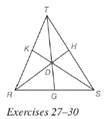Chapter 5.6, Problem 30EElementary Geometry For College St...

7th Edition
Alexander + 2 others
ISBN: 9781337614085

Solutions

Chapter
SectionElementary Geometry For College St...

7th Edition
Alexander + 2 others
ISBN: 9781337614085
Textbook Problem

Given point D in the interior of Δ R S T , suppose that R G = 2 , G S = 3 , S H = 3 and H T = 4. Find K T K R .To determine

To find:

The value of KTKR.

Explanation

Given:

Consider a point D in the interior of ΔRST and RG=2,GS=3,SH=3,HT=4.

Theorem used:

Ceva’s theorem:

Let point D be any point in the interior of ΔABC, where E, F, and G lie on ΔABC, let BE¯,AF¯ and CG¯ be the line segments determined by D and vertices of ΔABC. Then the product of the ratios of the lengths of the segments of each of the three sides (taken in order from a given vertex of the triangle) equals 1; that is AGGBBFFCCEEA=1.

Calculation:

Let D be a point in the interior of ΔRST.

By Ceva’s theorem, we get RGGSSHHTKTKR=1

Still sussing out bartleby?

Check out a sample textbook solution.

See a sample solution

The Solution to Your Study Problems

Bartleby provides explanations to thousands of textbook problems written by our experts, many with advanced degrees!

Get Started

Intervals Graph the set. 64. [4, 6) [0, 8)

Precalculus: Mathematics for Calculus (Standalone Book)

Evaluate the indefinite integral. 5tsin(5t)dt

Single Variable Calculus: Early Transcendentals, Volume I

Let f be the function defined by f(x) = 2x + 5. Find f(a + h), f(a), f(a2), f(a 2h), and f(2a h).

Applied Calculus for the Managerial, Life, and Social Sciences: A Brief Approach

The slope of the line tangent to the circle x2 + y2 = 100 at the point (6, 8) is: a) 34 b) 34 c) 43 d) 43

Study Guide for Stewart's Single Variable Calculus: Early Transcendentals, 8th